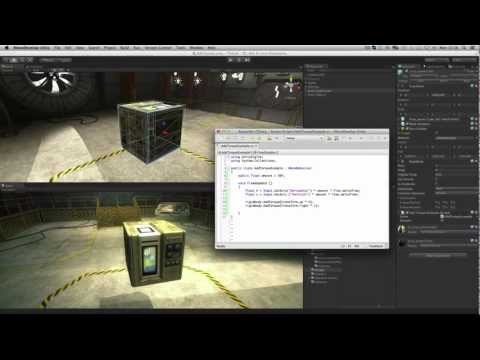# Adding Physics Torque

#### Dificuldade: Principiante

How to use Add Torque to rotate physics (rigidbody) objects.## Adding Physics Torque

Principiante Physics

Transcrições

### Code snippet

``````using UnityEngine;
using System.Collections;

public class AddTorqueExample : MonoBehaviour
{
public float amount = 50f;

void FixedUpdate ()
{
float h = Input.GetAxis("Horizontal") * amount * Time.deltaTime;
float v = Input.GetAxis("Vertical") * amount * Time.deltaTime;

rigidbody.AddTorque(transform.up * h);
rigidbody.AddTorque(transform.right * v);
}
}``````
``````#pragma strict

public var amount : float = 50f;

function FixedUpdate ()
{
var h : float = Input.GetAxis("Horizontal") * amount * Time.deltaTime;
var v : float = Input.GetAxis("Vertical") * amount * Time.deltaTime;

rigidbody.AddTorque(transform.up * h);
rigidbody.AddTorque(transform.right * v);
}``````
``````import UnityEngine
import System.Collections

public class AddTorqueExample(MonoBehaviour):

public amount as single = 50.0F

private def FixedUpdate():
h as single = ((Input.GetAxis('Horizontal') * amount) * Time.deltaTime)
v as single = ((Input.GetAxis('Vertical') * amount) * Time.deltaTime)

rigidbody.AddTorque((transform.up * h))
rigidbody.AddTorque((transform.right * v))``````

#### Documentação relacionada